• AHP层次分析法计算权重、特征值、特征向量，第一作者刘兴，其他地方下载需要47个币 【 尚有疑问，欢迎沟通！！ 1.CSDN上有人说可用； 2.亲自试验，代码流畅，但个人感觉特征值、特征向量存疑； 3.用java求出的特征...
• 使用c语言求矩阵的特征值和特征向量。使用数值积分方法，纯c语言编写，主要是用的QR分解，vs工程文件。
• 利用第三方矩阵计算工具包JAMA，求特征值、特征向量 API： http://math.nist.gov/javanumerics/jama/doc/Jama/Matrix.html 应用： 1.JAMA包的导入 1）https://mvnrepository.com/ 2）搜索JAMA，选第一个：gov....
• 特征值计算的新特征向量 该存储库实现了此新，该使我们能够通过PyTorch从特征向量优雅地计算出特征向量。 我将其移植到PyTorch，因为我的许多工作流程都在使用PyTorch的GPU上。 在Google Colab上运行Notebook 作者...
• 主要为大家详细介绍了C++ Eigen库计算矩阵特征值及特征向量，具有一定的参考价值，感兴趣的小伙伴们可以参考一下
• 颜庆津版数值分析编程作业，C语言(少量C++语法)实现矩阵的QR分解法迭代求矩阵的全部复数格式特征值，先对矩阵进行拟上三角化再迭代，迭代求出全部特征值后使用列主元素高斯消元法求出所有实特征值对应的特征向量
• 今天小编就为大家分享一篇Pytorch提取模型特征向量保存至csv的例子，具有很好的参考价值，希望对大家有所帮助。一起跟随小编过来看看吧
• 提取任何图像的特征向量，并找到余弦相似度以使用Pytorch进行比较。 我已经使用ResNet-18提取图像的特征向量。 最后，开发了一个Django应用程序来输入两个图像并找到余弦相似度。 包装方式： 火炬 Django 2.0 学分...
• 在使用AHP(层次分析法)时，需要计算特征值，使用pyhon读取Excel数据处理 此程序使用python读excel数据计算特征值和特征向量； 使用了numpy、xlrd、xlwt库；
• 《矩阵特征值与特征向量的定义与性质》教学设计.pdf
• 深入整理了雅克比方法求解特征值和特征向量，包括公式推导，最后介绍了opencv中的算法流程和实现。
• 经典jacobi法求解特征值特征向量，MATLAB代码实现。。MATLAB
• 计算矩阵特征值，采用幂法求矩阵的主特征值及主特征向量
• 给出基于多特征向量效益评估的评价框架，选取电力交易评价指标体系，根据指标体系确定综合评价指标集和评价集，采用灰色关联度综合评价方法描述一个信息矩阵中各个指标因素间相对变化的情况，利用多特征向量效益评估...
• 采用数值分析中的反幂法来求解矩阵的特征值，采用matlab实现
• 幂法求矩阵特征值特征向量matlab程序，但是区别于matlab自带方法。
•文档
• 通过用vba求矩阵的特征值与特征向量。可用于大多数
• 本代码用C语言进行编程，可以计算矩阵的特征值和相应的特征向量
• 雅克比法求取矩阵特征值和特征向量 考虑线性方程组Ax = b时，一般当A为低阶稠密矩阵时，用主元消去法解此方程组是有效方法。但是，对于由工程技术中产生的大型稀疏矩阵方程组，利用迭代法求解此方程组就是合适的，在...
• 下面以Matlab为例进行讲解求取矩阵特征值和特征向量求解。（Python和C/C++的相应代码在博客的最后给出）当一个计算机专业的人放下手头的工作，转而写博客时。说明他的CSDN账号积分所剩无几了。。。。。。基于Matlab...
下面以Matlab为例进行讲解求取矩阵特征值和特征向量求解。（Python和C/C++的相应代码在博客的最后给出）
当一个计算机专业的人放下手头的工作，转而写博客时。说明他的CSDN账号积分所剩无几了。。。。。。

基于Matlab为例进行讲解求取矩阵特征值和特征向量求解的具体步骤如下：

步骤一：确定所求矩阵

X = [2 1 0;1 3 1;0 1 4];

步骤二：求解X的特征值和特征向量
[V,D] = eig(X)

步骤三：运行结果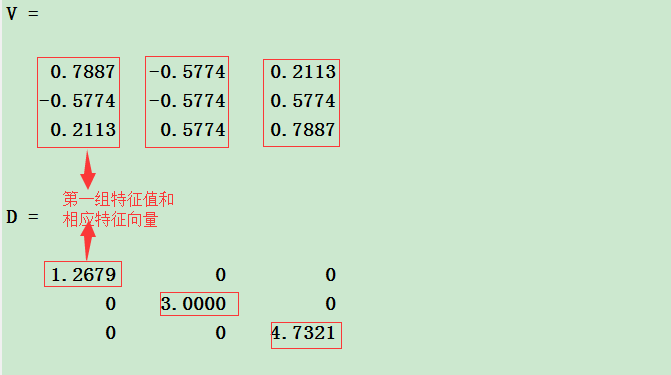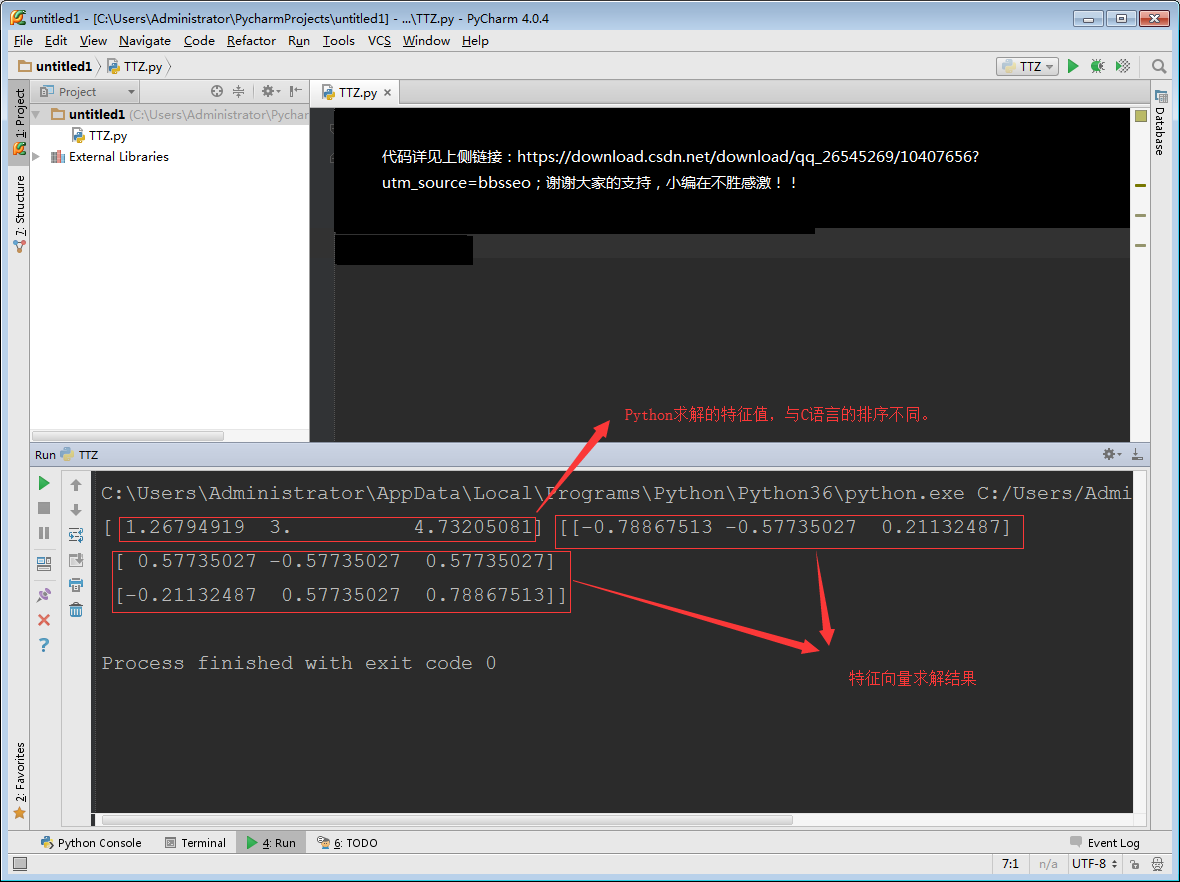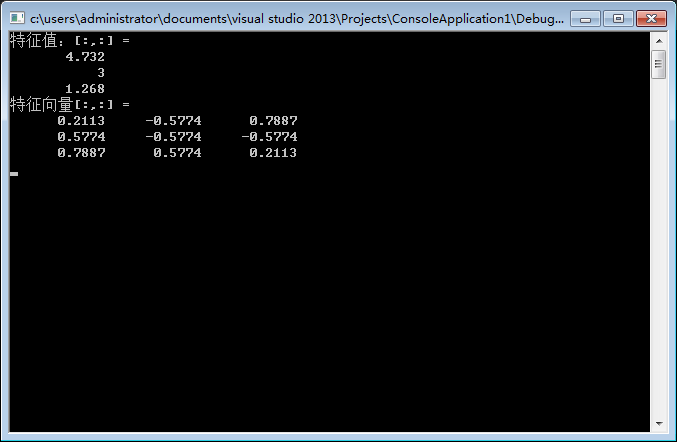展开全文• 本代码用opencv实现提取图像SIFT特征向量
• 已知矩阵A，在MATLAB界面输入[x,y]=eig(A)，可以得到特征值以及特征向量。其中y为对角阵，每个元素为特征值；x的每一列为特征值所对应的特征向量。 需要进行特征向量标准化时，可以输入x(:,1)/norm（x(:,1)）将特征...
已知矩阵A，在MATLAB界面输入[x,y]=eig(A)，可以得到特征值以及特征向量。其中y为对角阵，每个元素为特征值；x的每一列为特征值所对应的特征向量。
需要进行特征向量标准化时，可以输入x(:,1)/norm（x(:,1)）将特征向量进行标准化，标准化的特征向量各个元素平方之和为1。
展开全文MATLAB
•  （由于这句话会引申出特别多的性质，所以本文就不举这句话的例子了） 3、给定一个矩阵，求特征值与特征向量的方式 求特征值与特征向量为这么一个过程：设A为n阶矩阵，a为非零列向量，λ是一个数， 4、特征值与特征...
一、线性代数的入门知识
（一）矩阵
1、矩阵的表示
在中学的时候，我们会经常看到这样子的方程组：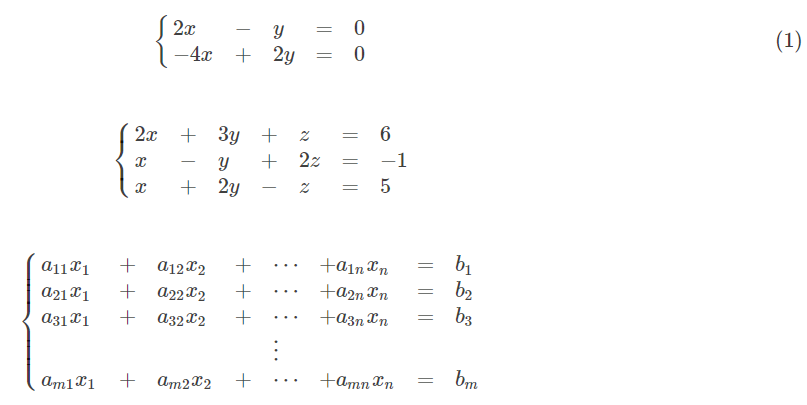看到这样子的方程组，不由感到十分怀念。不过有没有这种感想，当年解三元一次方程组的时候，特别烦，消元后要抄一遍，代入后又抄一遍，特别麻烦。
于是数学家发明了矩阵，把方程组中所有系数写到了一个框里面，把所有未知数写到第二个框里，把所有等式右边的值写到第三个框里。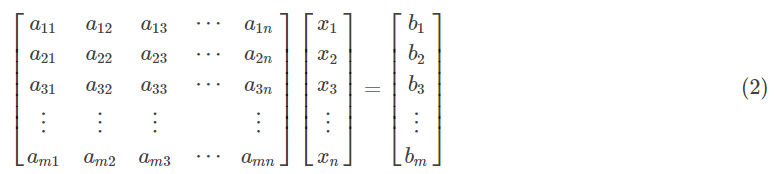比如方程组 (1) 也可表示为：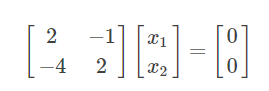观察 (2) 式不难发现，复杂的方程组用矩阵表示后，还是很复杂，所以可以把 (2) 式更加简洁地表示成增广矩阵：同理，比如方程组 (1) 也可表示为增广矩阵：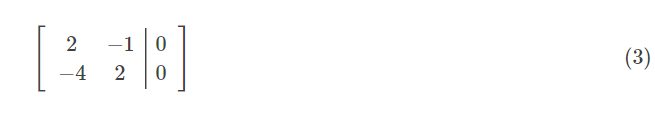特别地，当方程组的等式右边全为0，即bi=0，其中i=1,2,3…n时，方程组为齐次线性方程组，增广矩阵可直接表示成系数矩阵。比如增广矩阵 (3) 可直接表示成：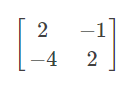我们称，方程组的等式右边全为0的方程组为齐次线性方程组，否则为非齐次线性方程组。

2、矩阵的解方程组
（1）齐次线性方程组
来回顾一下这个方程组：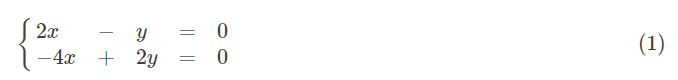等式右边全为0，所以把这个方程组写成矩阵形式：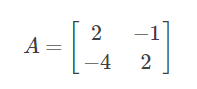要解这个方程组，当然使用消元法，不同于中学的是直接在矩阵里面消元：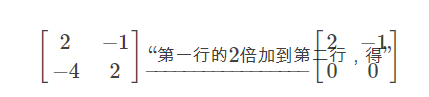看到消元后的新矩阵是不是觉得很直观，如果你你把新矩阵还原成方程组的形式，有：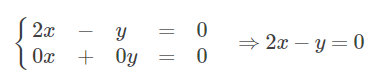仔细观察可发现，原本有两个式子的方程组经过消元后，变成了只有一个方程组。这种情况在中学时，无论做多少题都不会遇到的，
因为在中学里，学的初等数学方程组都是有唯一解的。而在线性代数中，我们把这种情况成为方程组系数矩阵的秩为1，记为r(A)=1。
当矩阵的秩小于未知数的个数时，方程组有无数个解；当矩阵的秩等于未知数的个数时，方程组只有零解。
由于方程组(1)有两个未知数，而r(A)=1<2，所以方程组(1)有无数个解。设 y=2 ,则 x=1；再设 k 为任意常数，则 x=k, y=2k 为方程组(1)的解，写成矩阵的形式为：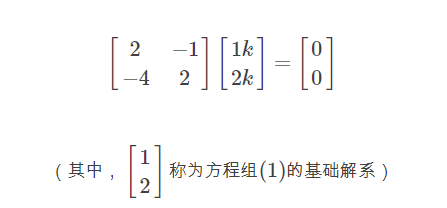（2）非齐次线性方程组
再来看一个3个未知数的方程组：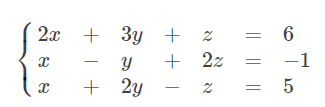右边等式不为0，改写成增广矩阵：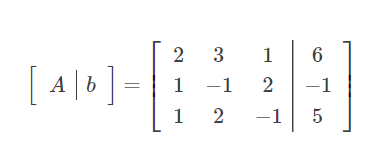同理，对 [ A | b] 进行初等行变换（即消元）：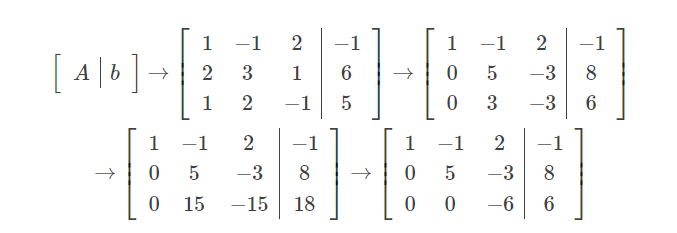这一次进行初等行变换后，对于任意的非齐次线性方程组，当 r(A)=r(A|b)=未知数的个数 时，非齐次线性方程组有唯一解；
当 r(A)=r(A|b)<未知数的个数 时，非齐次线性方程组有无数个解；当 r(A) 不等于 r(A|b) 时，非齐次线性方程组无解。
可见 r(A)=r(A|b)=3，所以[A|b]有唯一解，写回方程组形式：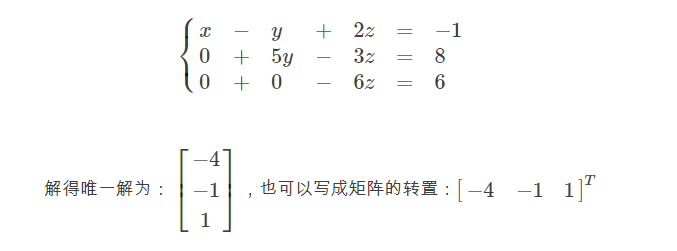（二）向量
（1）列向量
可能是数学家觉得用矩阵来代表方程组还是太麻烦还废纸，所以又苦思冥想，最终想到了用向量再来继续简化矩阵，举个例子：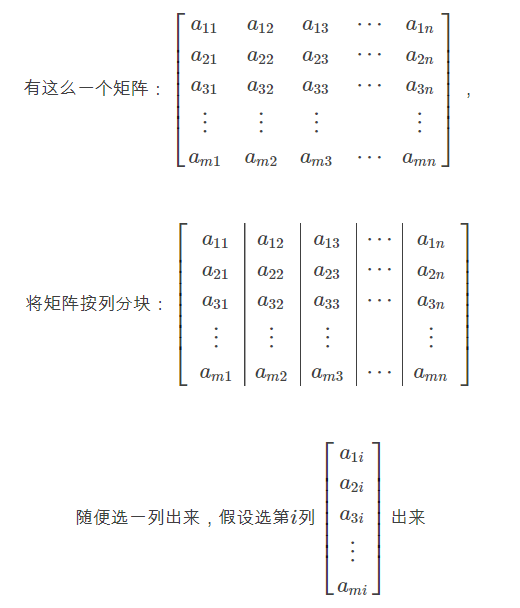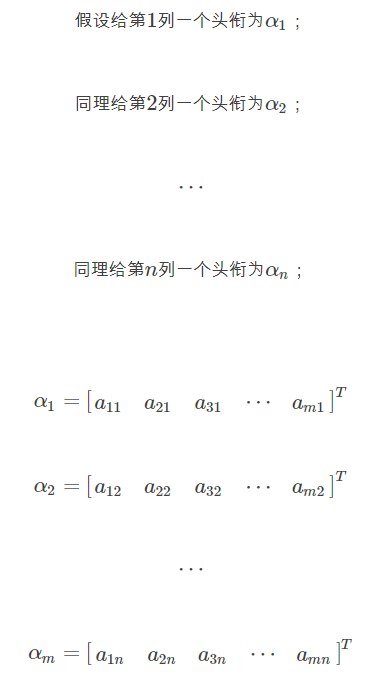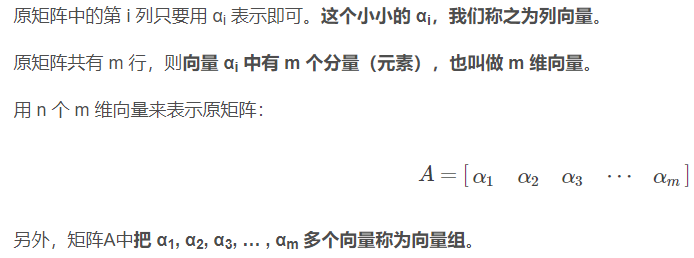（2）行向量
有列向量，自然有行向量。同理，我们对矩阵A按行分块，并对每行元素用向量表示。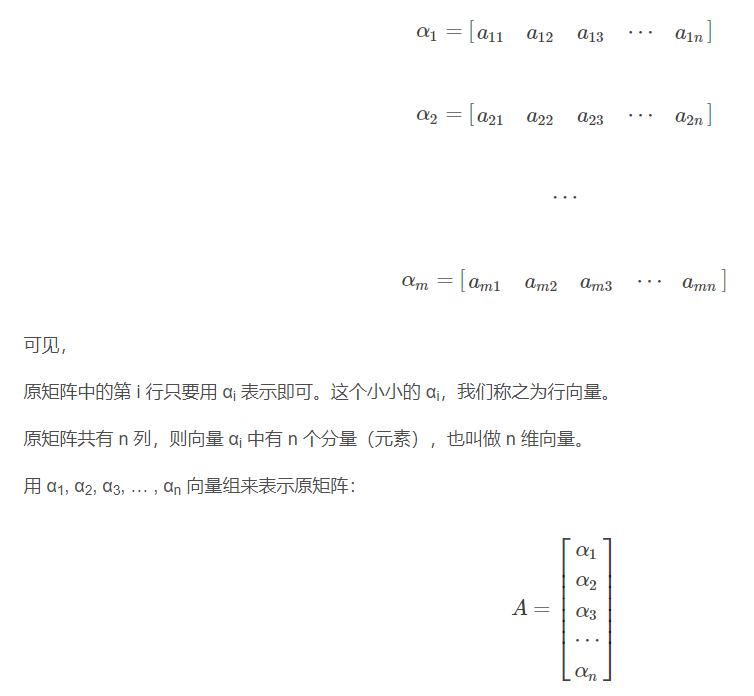显然，用向量组便可轻松对矩阵瘦身。

3、线性相关与线性无关
（1）线性相关
假设有这么一个矩阵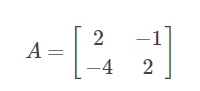我们对其按行分块，则有向量：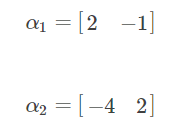如果用行向量 α1, α2, α3, … , αn 来表示的矩阵A经过初等行变换后，某行（即某个向量αi）的元素全变为0，
那么则称 α1, α2, α3, … , αn 向量组线性相关（可以理解为多个向量间或系数矩阵有线性关系）。
如果齐次线性方程组的系数矩阵有线性关系，那么齐次线性方程组有无穷多个解。了看清楚 α1, α2, α3, … , αn 是否线性相关，对矩阵A进行初等行变换后，发现：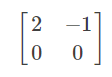所以， α1, α2, α3, … , αn 向量组线性相关
（2）线性无关
假设有这么一个矩阵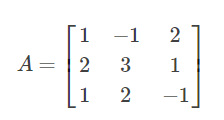我们对其按行分块，则有向量：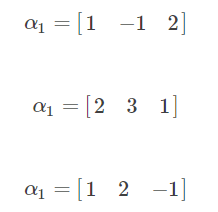如果用行向量 α1, α2, α3, … , αn 来表示的矩阵A经过初等行变换后，某行（即某个向量αi）的元素不全为0，那么则称 α1, α2, α3, … , αn 向量组线性无关。
为了看清楚 α1, α2, α3, … , αn 是否线性无关，对矩阵A进行初等行变换后，发现：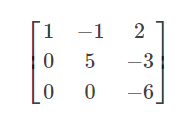所以， α1, α2, α3, … , αn 向量组线性无关。

4、向量空间
向量空间是线性代数中抽象的一部分，常用于物理研究中探索多维空间的奥秘（有没有用于物理中不太清楚，感觉的）。
在笛卡尔直角坐标体系中，向量(1,0), (0,1)分别代表了横坐标轴、纵坐标轴，对这两个向量线性组合的整体就可以表示出一个平面，即2维向量空间；
向量(1,0,0), (0,1,0), (0,0,1)分别代表了x坐标轴、y坐标轴、z坐标轴，对这三个向量线性组合表示出的整体可表示出一个3维向量空间；以此类推。
有规律的是，n 维向量空间中的 n个坐标轴向量是互相垂直的（就算是4维空间的4个坐标轴也是垂直的，只不过我们处于三维中，难以感知到四维空间，只能想象）。
我们称向量间的垂直为正交。数学家对向量空间更是大开脑洞，认为不一定是笛卡尔体系的(1,0,0), (0,1,0), (0,0,1)才能是坐标轴，
只要是线性无关的向量组中的n个向量都可以当做是n维向量空间中的坐标轴，相当于将笛卡尔坐标体系的原点固定住，
将所有坐标轴就像陀螺一样旋转某个角度，得出的新坐标轴肯定是线性无关的。
“n个向量组成的线性无关向量组，在n维向量空间中，可充当坐标轴” 这就是线性代数的向量空间的核心思想。

（三）行列式

行列式作为国内各个教材书中的第一个章节的内容，证明了其在线性代数中的重要性。但是如果从教材中的行列式入手学习线性代数，
那是要吃不少苦头的，因为只学了行列式，没有具备矩阵和向量的知识的情况下，很容易一脸懵逼。
由于行列式涉及的概念、性质、计算众多，所以本文只简单介绍一下行列式。

行列式的本质是什么？柯西给出了答案。假设在一个平面中有两个向量：x1=(a, c), x2=(b, d)；
x1与横坐标的角度为α，x2与横坐标的角度为β；
x1的模为 ||x1||，x2的模为 ||x2||；  如图：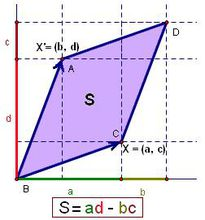我们要求图中的 S，即向量平移后端点相交而围成的平行四边形的面积：
S = ||x1|| · ||x2|| · sin(β-α)
= ||x1|| · ||x2|| · (sinβcosα - cosβsinα)
对 x1 和 x2 向量类似行向量那样子对元素命名：
x1=(a11, a12)
x2=(a21, a22)
重新得到 S = a11 · a22 - a12 · a21，
数学家对向量 x1 和 x2 写成行列式，代表了 S 的值：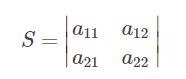行列式不过就是将矩阵的括号改成了两条竖线，用竖线包着的元素，最终可以算出一个数，这个数就是行列式，行列式就是一个数，
这个数是不同行不同列元素乘积的代数和。而矩阵本质为表格或数组，这是两者不同之处。
如果继续推，可以推出：在二维空间，行列式是面积；在三维空间，行列式是体积；在高维空间，行列式是一个数。
换句话说，就是由n个向量组成的线性无关向量组，可以表示成行列式，并且算出的结果肯定不为0。
以次可推，由n个向量组成的线性相关向量组表示成的行列式值为0。
（四）特征值与特征向量

特征值与特征向量在线性代数中是最难理解的内容，尤其在阅读国内教程时，直接一个定义拍到脸上，让人措手不及。
特征值与特征向量是线性代数的核心，在机器学习算法中应用十分广泛。

1、定义
首先，讲到特征值与特征向量，必先讲到定义：设 A 是 n 阶矩阵，如果存在一个数 λ 及非零的 n 维列向量 α ，使得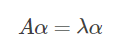成立，则称 λ 是矩阵 A 的一个特征值，称非零向量 α 是矩阵 A 属于特征值 λ 的一个特征向量。
观察这个定义可以发现，特征值是一个数，特征向量是一个列向量，一个矩阵乘以一个向量就等于一个数乘以一个向量。这个定义感觉太抽象了，我们来举一个具体的例子：
设 A 是 3 阶矩阵，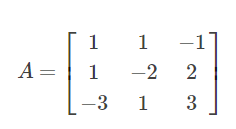存在一个数 λ=4 ，
且存在一个非零的 3 维列向量 α ，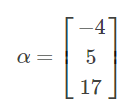使得 Aα = λα，即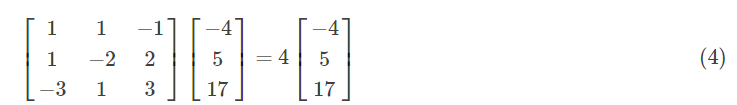则称 λ=4 为矩阵A的特征值，（为了方便，简称 4 为特征值）；也称 α=[ -4, 5, 17 ]T 是矩阵A属于特征值为 4 的一个特征向量。
（为了方便，简称 [ -4, 5, 17 ]T 为特征向量）
对于上面的(4)式，我们可以把它还原为方程组验证此式是否成立，还原过程如下：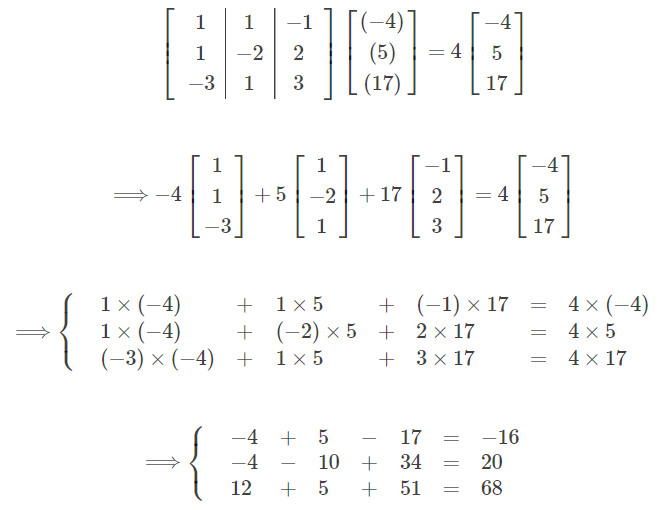显然，每个等式两边相等， Aα = λα 成立！（如果不知道为什么可以还原成方程组的话，请翻回到上面的非齐次方程组部分，可发现在这里 α 相当于解向量， λα 相当于 b 向量）

2、特征值与特征向量的个数 如果是自学线性代数的话，很容易有这么一个误区：认为一个矩阵的特征值与特征向量只有一个。
其实，一个矩阵的特征值可以有多个，相应地，特征向量的个数也随着特征值的数量的变化而变化。
总的来说，一个n行n列的矩阵的特征值个数少于或等于 n 个。还是以矩阵A为例，满足 Aα = λα 的式子有：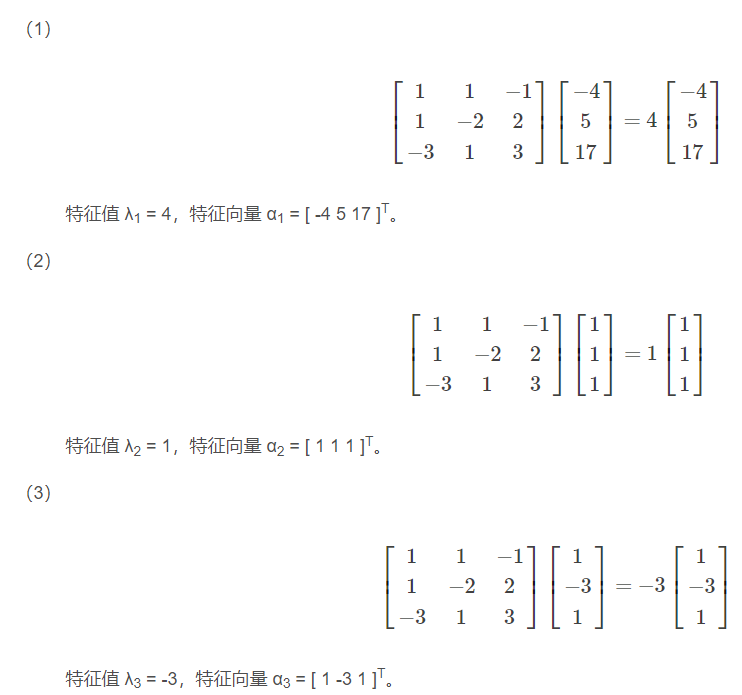另外，一个特征值对应的特征向量的个数也不一定只有一个。 （由于这句话会引申出特别多的性质，所以本文就不举这句话的例子了）

3、给定一个矩阵，求特征值与特征向量的方式
求特征值与特征向量为这么一个过程：设A为n阶矩阵，a为非零列向量，λ是一个数，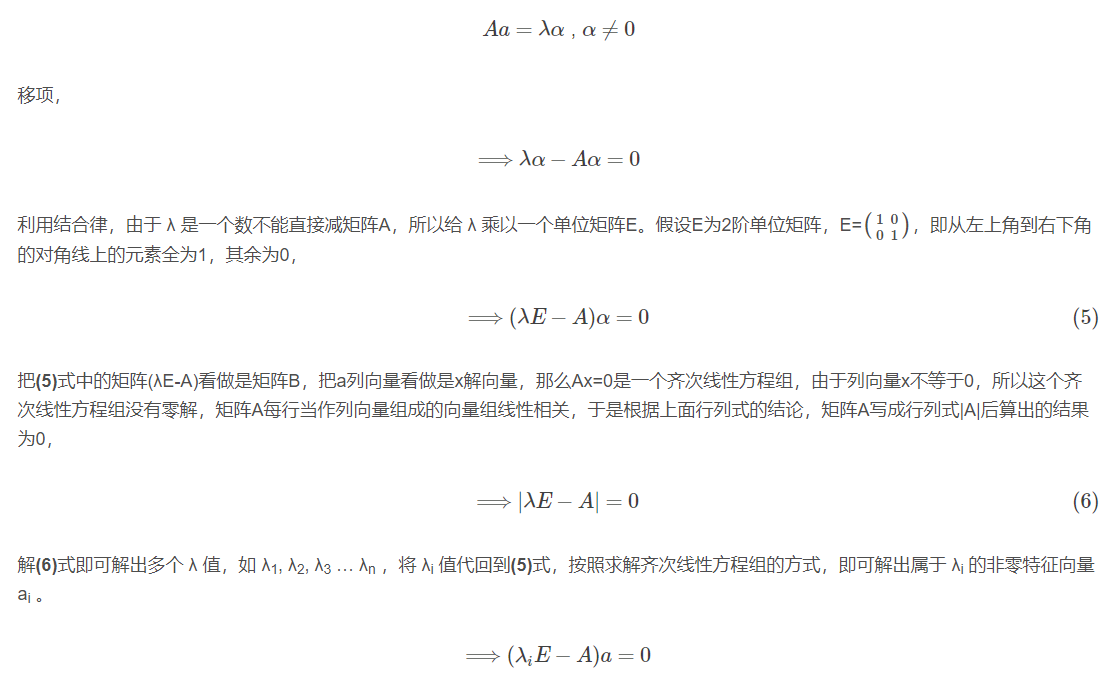4、特征值与特征向量该怎么理解
仔细看本文的童鞋就会发现，一个矩阵并非只是单纯的一张表格、数组，它还代表了某种神奇的魔力，
与某个向量相乘后，还能变成一个数。换句话说，保存着很多个数的矩阵经过与特定的向量相乘后，塌缩成了一个数。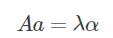对于特征值与特征向量，有许多不同的理解，我自己从网络上的观点总结了一下，大概分为三种理解：
第一种理解：从向量的角度来看，一个列向量在左乘一个矩阵后，会经过一系列的线性变换，最终向量的长度会变成原来的 λ 倍。
第二种理解：从矩阵的角度来看，矩阵是一种线性变化的描述，特征向量是一个不变的方向，特征值是线性变化的结果。
第三种理解：从向量空间的角度来看，因为不同特征值对应的特征向量线性无关，把每个特征向量看做是一个坐标轴，
特征值是对应坐标轴（即特征向量）的坐标值。简单来说，就是用特征值（坐标）与特征向量（坐标轴）来表示原矩阵。
以上三种理解由浅入深，第三种理解才是本质的理解，但首先需要对向量空间有深刻的理解。


展开全文• 矩阵特征值和特征向量解法的研究.doc，对矩阵特征值和特征向量的一些方法进行了系统的归纳和总结.在比较中能够 更容易发现最好的方法，并提高问题的解题效率.
• 二、举个例子1、计算特征值与特征向量2、用特征向量表示任意向量三、理解其他结论1、对角化分解2、矩阵的特征值分别是原矩阵特征值的倒数3、特征值为0,意味着不可逆4、通过解Ax⃗=λx⃗A\vec{x}=\lambda \vec{x}Ax=...


特征值和特征向量的理解
前言一、矩阵是什么？二、举个例子1、计算特征值与特征向量2、用特征向量表示任意向量
三、理解其他结论1、对角化分解2、矩阵的特征值分别是原矩阵特征值的倒数3、特征值为0,意味着不可逆4、通过解

A

x

⃗

=

λ

x

⃗

A\vec{x}=\lambda \vec{x}

来寻找特征值

前言
本文将探讨线性代数中及其重要的两个概念:特征值与特征向量.

提示：(PS:下文中的矩阵A均认为是方阵) 。
一、矩阵是什么？
矩阵不单单是二维数组，它更重要的角色是映射：

y

⃗

=

A

x

⃗

\vec{y}=A\vec{x}

y

⃗

=

A

x

⃗

\vec{y}=A\vec{x}

就相当于

y

⃗

=

f

(

x

⃗

)

\vec{y}=f(\vec{x})

,矩阵A是把向量

x

⃗

\vec{x}

映射到向量

y

⃗

\vec{y}

的一个函数,或者说,映射,算子。
从一般的角度看,这个映射无非就是矩阵乘向量,说得具体一点,就是n次的向量点积计算.(矩阵的一行乘上向量,并对结果向量的所有元素求和,就是一次点积)
错!实际上,这个映射本质是一个缩放操作.
二、举个例子
1、计算特征值与特征向量
提示：可以动动手指头算一算，参考这里。
举一个简单的例子,矩阵

A

=

(

4

−

2

3

−

1

)

A= \left( \begin{array}{ccc} 4 & -2 \\ 3 & -1 \end{array} \right)

它的特征值分别是2和1,特征向量是

(

1

1

)

\left( \begin{array}{ccc} 1 \\ 1 \end{array} \right)

和

(

2

3

)

\left( \begin{array}{ccc} 2 \\ 3 \end{array} \right)

2、用特征向量表示任意向量
我们随便设向量

x

⃗

=

(

1

2

)

\vec{x}=\left( \begin{array}{ccc} 1 \\ 2 \end{array} \right)

，显然结果

y

⃗

=

A

x

⃗

=

(

0

1

)

\vec{y}=A\vec{x}=\left( \begin{array}{ccc} 0 \\ 1 \end{array} \right)

我们使用另一种方法计算,首先我们将

x

⃗

\vec{x}

表示成特征向量

(

1

1

)

\left(\begin{array}{ccc} 1 \\ 1 \end{array} \right)

和

(

2

3

)

\left( \begin{array}{ccc} 2 \\ 3 \end{array} \right)

的线性组合，即：

x

⃗

=

(

1

2

)

=

−

1

∗

(

1

1

)

+

1

∗

(

2

3

)

\vec{x}=\left( \begin{array}{ccc}1\\2\end{array} \right)=-1*\left( \begin{array}{ccc}1\\1\end{array} \right) + 1* \left( \begin{array}{ccc}2\\3\end{array} \right)

然后,我们将特征值和对应的系数相乘,得到:

y

⃗

=

−

1

∗

2

∗

(

1

1

)

+

1

∗

1

∗

(

2

3

)

=

−

2

∗

(

1

1

)

+

1

∗

(

2

3

)

\vec{y}=-1*2*\left( \begin{array}{ccc}1\\1\end{array} \right) + 1*1* \left( \begin{array}{ccc}2\\3\end{array} \right)=-2*\left( \begin{array}{ccc}1\\1\end{array} \right) + 1* \left( \begin{array}{ccc}2\\3\end{array} \right)

显然,如果你继续计算下去,你也会得到

y

⃗

=

(

0

1

)

\vec{y}=\left( \begin{array}{ccc}0\\1\end{array} \right)

提示：好好领悟
特征值和特征向量的意义就在于此! 矩阵所充当的映射,实际上就是对特征向量的缩放,每个特征向量的缩放程度就是特征值. 因此,我们需要将向量

x

⃗

\vec{x}

表示成特征向量的线性组合(相当于以特征向量为基),得到相应的特征向量的权重.
然后,每个权重与特征值相乘,就是这个映射最本质的缩放操作.
三、理解其他结论
基于这样的理解,我们可以很简单地解释很多结论。
1、对角化分解
对角化分解实际上就是我们解释特征值含义的过程。

A

=

P

Λ

P

−

1

A=P\Lambda P^{-1}

,其中

P

P

是由特征向量组成的矩阵,

Λ

Λ

是由特征值组成的对角矩阵。

在解释对角化分解之前,我们还要先解释矩阵的另一个含义. 对于

z

⃗

=

P

y

⃗

\vec{z}=P\vec{y}

, 事实上矩阵P还有其他含义,比如在这里有转换基向量的含义:

y

⃗

\vec{y}

是特征向量为基所表示的向量，上文

y

⃗

=

−

2

∗

(

1

1

)

+

1

∗

(

2

3

)

\vec{y}=-2*\left( \begin{array}{ccc}1\\1\end{array} \right) + 1* \left( \begin{array}{ccc}2\\3\end{array} \right)

，那么

y

⃗

\vec{y}

在在特征向量为基的表示是

y

⃗

=

(

−

2

1

)

\vec{y}=\left( \begin{array}{ccc}-2\\1\end{array} \right)

z

⃗

\vec{z}

则是坐标轴为基所表示的向量,假如

z

⃗

\vec{z}

的表示为

z

⃗

=

(

0

1

)

\vec{z}=\left( \begin{array}{ccc}0\\1\end{array} \right)

，相当于

z

⃗

=

0

∗

(

1

0

)

+

1

∗

(

0

1

)

\vec{z}=0*\left( \begin{array}{ccc}1\\0\end{array} \right) + 1* \left( \begin{array}{ccc}0\\1\end{array} \right)

那么

z

⃗

=

P

y

⃗

\vec{z}=P\vec{y}

的含义就是把一个向量从特征向量为基的表示

y

⃗

\vec{y}

,转变成以坐标轴为基的表示

z

⃗

\vec{z}

. 相应,

y

⃗

=

P

−

1

x

⃗

\vec{y}=P^{-1}\vec{x}

的含义则相反,是把一个向量从坐标轴为基的表示

x

⃗

\vec{x}

,转变成以特征向量为基的表示

y

⃗

\vec{y}

.

那么

y

⃗

=

A

x

⃗

=

P

Λ

P

−

1

x

⃗

\vec{y}=A\vec{x}=P\Lambda P^{-1} \vec{x}

，我们就可以解释了。 首先,

P

−

1

x

⃗

P^{-1}\vec{x}

是将

x

⃗

\vec{x}

转变成用特征向量表示,上文

x

⃗

=

(

1

2

)

=

−

1

∗

(

1

1

)

+

1

∗

(

2

3

)

\vec{x}=\left( \begin{array}{ccc}1\\2\end{array} \right)=-1*\left( \begin{array}{ccc}1\\1\end{array} \right) + 1* \left( \begin{array}{ccc}2\\3\end{array} \right)

，就是把

(

1

2

)

\left( \begin{array}{ccc} 1 \\ 2 \end{array} \right)

变成了

(

−

1

1

)

\left( \begin{array}{ccc} -1 \\ 1 \end{array} \right)

。 然后

Λ

P

−

1

x

⃗

\Lambda P^{-1} \vec{x}

,就是对应的特征值与权重作乘法,得到

(

−

2

1

)

\left( \begin{array}{ccc} -2 \\ 1\end{array} \right)

。 最后

y

⃗

=

P

Λ

P

−

1

x

⃗

\vec{y}=P\Lambda P^{-1} \vec{x}

，就是把

(

−

2

1

)

\left( \begin{array}{ccc} -2 \\ 1\end{array} \right)

重新转换成坐标轴为基的表示,得到

(

0

1

)

\left( \begin{array}{ccc} 0 \\ 1\end{array} \right)

。
2、矩阵的特征值分别是原矩阵特征值的倒数
是因为原矩阵放大了2倍,逆矩阵就要相应地缩小到原本的1/2.
当然,特征向量要保持对应,因此这也解释了为什么逆矩阵的特征向量和原矩阵一样
3、特征值为0,意味着不可逆
参考第2点,0没有倒数.
4、通过解

A

x

⃗

=

λ

x

⃗

A\vec{x}=\lambda \vec{x}

来寻找特征值
显然,在这里λ是特征值,

x

⃗

\vec{x}

是特征向量.
把

x

⃗

\vec{x}

变成以A的特征向量为基来表示的话,那么权重肯定只有1个1,其他都为0,那个1对应的特征向量当然是

x

⃗

\vec{x}

本身.
这个时候进行缩放,那么只有

x

⃗

\vec{x}

的权重被缩放了,其他特征向量的权重都是0,0乘任何数为0.
那么,A

x

⃗

\vec{x}

的结果就相当于

λ

x

⃗

\lambda\vec{x}

了,因为

λ

x

⃗

\lambda\vec{x}

就是

x

⃗

\vec{x}

作了相应的缩放,缩放因子就是特征值λ.
展开全文线性代数 矩阵
• 论文研究-梯度特征向量排序法的推导与改进.pdf, 层次分析法作为多准则决策的一种实用方法,近年来有了迅速发展,本文介绍的特征向量法能较好地解决层次分析法中所遇到的判断矩阵很不一致或有明显偏好性时求权向量的...
• 生成具有正态分布的2D数据。 数据被移位和旋转。 然后，将SVD用于计算特征向量和特征值。 特征向量和特征值的物理含义可以通过其图来说明。matlab
• 这几天做一个项目，需要用到 求矩阵的特征值特征向量。我c++学的不好，所以就去网站找了很多java的源代码，来实现这个功能。很多都不完善，甚至是不准确。所以自己参考写了一个。这个用于我一个朋友的毕业设计。结果......